<input id="ohw05"></input>
• <var id="ohw05"></var>
• <code id="ohw05"><cite id="ohw05"></cite></code>
<label id="ohw05"></label>
<var id="ohw05"></var>

# 接雨水系列問題

作者：Grey

原文地址：接雨水系列問題

## LeetCode 42. 接雨水

主要思路：考慮每個位置，頂部可以留下多少水，累加起來，就是總的接水量。

其中，最右側和最左側的頂部無法接到水，因為水會從兩側流走。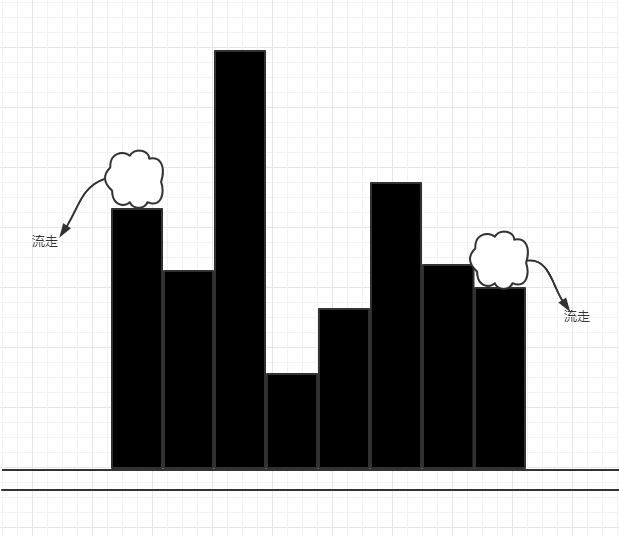基于上述邏輯，至少可以判斷，如果數組的長度小于等于2，直接返回0份水。

當數組的長度大于2，我們需要考慮，從1號位置到數組長度-2，每個位置頂部能接多少水。

設置四個變量

        int l = 1;
int r = arr.length - 2;
// 左側目前高度的瓶頸是多少
int lMax = arr;
// 右側目前高度的瓶頸是多少
int rMax = arr[arr.length - 1];


lMaxrMax作為左側和右側的在當前狀態下的瓶頸，我們實際遍歷的位置從lr，看下arr[l]arr[r]位置基于左右側的瓶頸能收集到的水量是多少。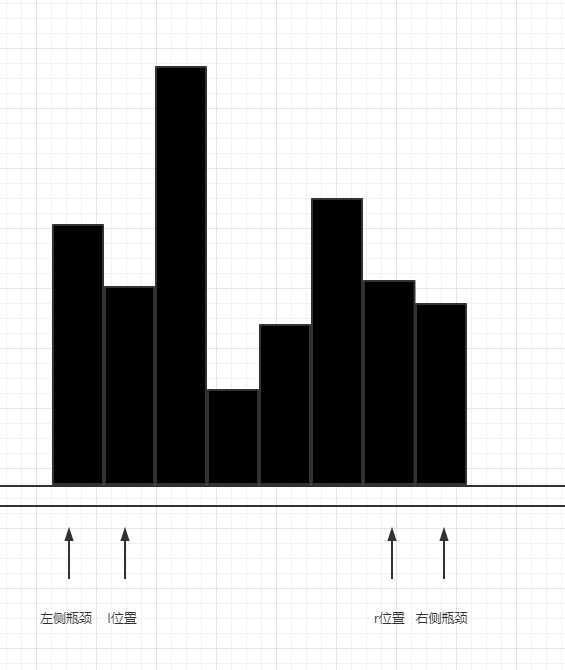首先，雖然有兩個瓶頸值，但是，我們在結算的時候，只能以較小的瓶頸值來結算，因為如果以較大瓶頸值來結算，小的瓶頸會拉低當前的結算值（木桶效應）。

所以，有兩種情況：

情況一：lMax > rMax

情況二：lMax <= rMax

在情況一下，例如在這種情況下，我們可以確定arr[r]位置上的水能接多少，因為arr[r]和右側瓶頸是確定的關系，左側瓶頸雖然比arr[r]大，是水會因為右側瓶頸讓arr[r]收集的水流走，所以此時

arr[r]上可以收集的水量就是arr[r]rMax之間的差值，如果是負數，則為0，相當于沒有收到水，此時，r位置遍歷完畢，右側瓶頸更新為Math.max(arr[r],rMax)，按上圖示例，現在的右側瓶頸變成了arr[r]上的值。如下圖在這種狀態下，相對小的瓶頸是rMax，又可以結算當前arr[r]位置的水量，還是0。然后繼續更新右側瓶頸，如下圖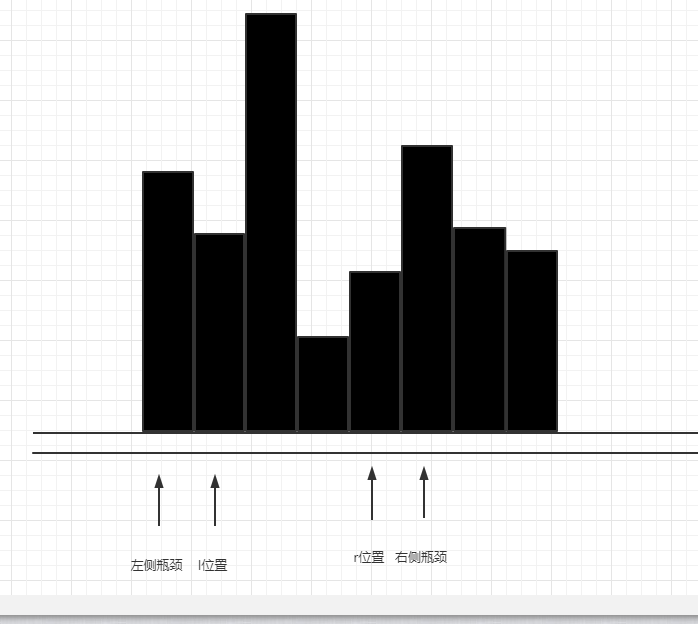此時，左側瓶頸是較小的那個，現在就要更新arr[l]頭頂上的水量，即：arr[l]lMax之間差值和0比較較大的那個，然后移動l指針，更新左側瓶頸（由于arr[l]值沒有lMax大，所以左側瓶頸保留在原先lMax位置），如下圖。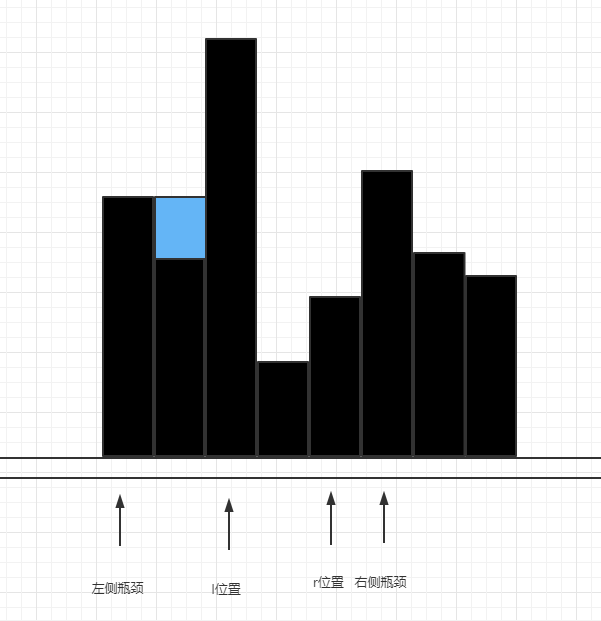接下來lMax < rMax，繼續結算arr[l]，移動l和更新lMax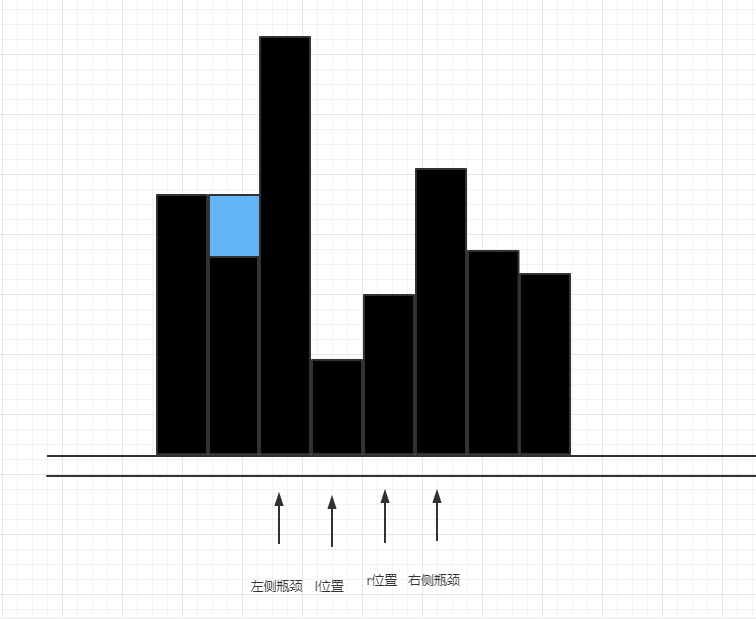接下來的過程同理，示意圖如下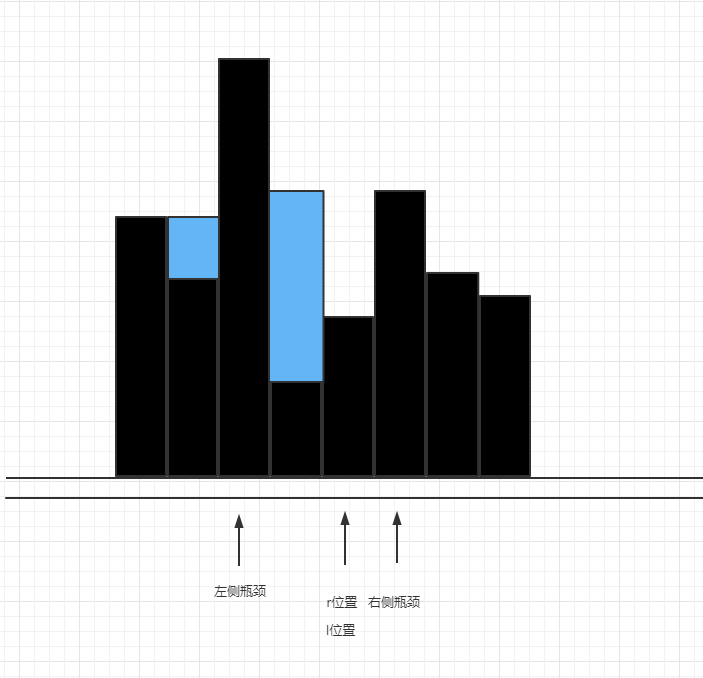直到l>r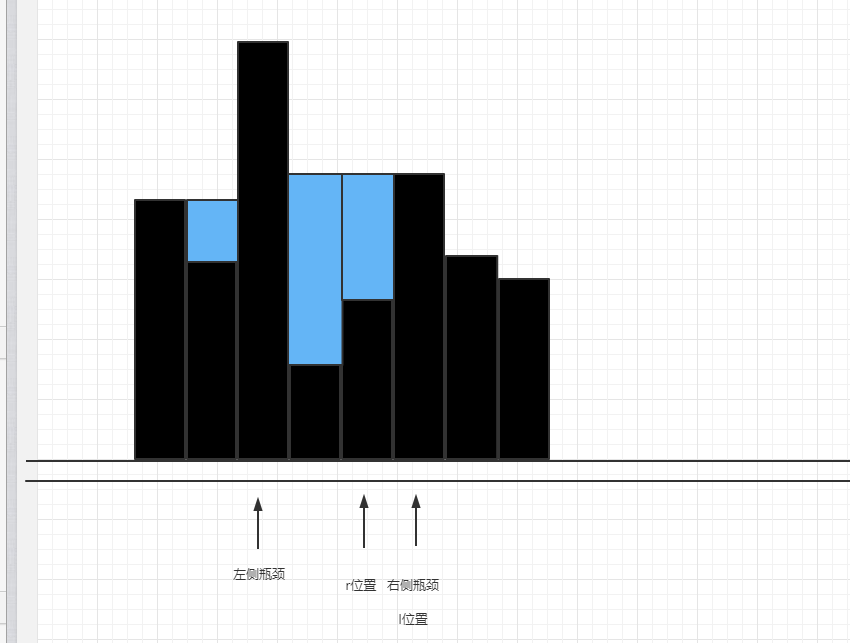所有水收集完畢。

完整代碼見

    public static int trap(int[] arr) {
if (arr == null || arr.length <= 2) {
return 0;
}
int result = 0;
int l = 1;
int r = arr.length - 2;
int lMax = arr;
int rMax = arr[arr.length - 1];
while (l <= r) {
if (lMax < rMax) {
// 結算左側
result += Math.max(0, lMax - arr[l]);
lMax = Math.max(lMax, arr[l++]);
} else {
// 結算右側
result += Math.max(0, rMax - arr[r]);
rMax = Math.max(rMax, arr[r--]);
}
}
return result;
}


時間復雜度O(N)，空間復雜度O(1)

## LeetCode 407. 接雨水 II

主要思路

和一維的思路一樣，二維接雨水問題也是先定位瓶頸，一維接雨水的瓶頸是從左右兩端來定位，二維的瓶頸就是從矩陣的四周來定位。要找到四周的最小瓶頸，我們需要用一個小根堆（按值組織），將四周的值加入小根堆，彈出的值即為最小瓶頸。首先，我們需要包裝一下原始矩陣，設計一個Node數據結構，用于存放原始矩陣中的某個值以及其位置信息。

    public static class Node {
public int v; // 值
public int i; // 行位置
public int j; // 列位置

public Node(int v, int i, int j) {
this.i = i;
this.j = j;
this.v = v;
}
}


小根堆里面存的就是Node，按照Nodev來組織，

PriorityQueue<Node> heap = new PriorityQueue<>(Comparator.comparingInt(o -> o.v));


每當彈出一個Node的時候，將這個位置的上下左右（先確保不越界）進行結算，結算完成后，將已經結算的位置加入小根堆（已經加入過的位置就不用重復加入了），由于需要知道哪些位置加入過小根堆，所以設置一個二維的boolean數組，用于標志某個位置是否進入過。

boolean[][] isEntered = new boolean[m][n];

if (isEntered[i][j]) {
// 某個位置進入過小根堆
} else {
// 否則沒有進入過
}


首先，我們將矩陣四周的點加入小根堆，并且把isEntered設置好

        // 以下兩個for循環，是把四周都加入小根堆
for (int i = 0; i < m; i++) {
heap.offer(new Node(heightMap[i], i, 0));
heap.offer(new Node(heightMap[i][n - 1], i, n - 1));
isEntered[i] = true;
isEntered[i][n - 1] = true;
}
for (int i = 0; i < n; i++) {
heap.offer(new Node(heightMap[i], 0, i));
heap.offer(new Node(heightMap[m - 1][i], m - 1, i));
isEntered[i] = true;
isEntered[m - 1][i] = true;
}


然后我們設置一個max，用于標識此時最小瓶頸是哪個。接下來的流程就是：

每次從小根堆彈出一個元素，看下能否更新瓶頸值，且看其四面八方的位置上是否越界，如果沒有越界，直接結算四面八方位置的值，累加到water這個變量中，同時把四面八方的Node加入小根堆，循環往復，直到小根堆為空。water的值即為收集到的水量。

完整代碼見

    public static int trapRainWater(int[][] heightMap) {
if (null == heightMap || heightMap.length <= 2 || heightMap.length <= 2) {
return 0;
}
int m = heightMap.length;
int n = heightMap.length;
int max = 0;
PriorityQueue<Node> heap = new PriorityQueue<>(Comparator.comparingInt(o -> o.v));
boolean[][] isEntered = new boolean[m][n];
// 以下兩個for循環，是把四周都加入小根堆
for (int i = 0; i < m; i++) {
heap.offer(new Node(heightMap[i], i, 0));
heap.offer(new Node(heightMap[i][n - 1], i, n - 1));
isEntered[i] = true;
isEntered[i][n - 1] = true;
}
for (int i = 0; i < n; i++) {
heap.offer(new Node(heightMap[i], 0, i));
heap.offer(new Node(heightMap[m - 1][i], m - 1, i));
isEntered[i] = true;
isEntered[m - 1][i] = true;
}
int water = 0;
while (!heap.isEmpty()) {
// 最薄弱的位置
Node weakest = heap.poll();
max = Math.max(weakest.v, max);
int i = weakest.i;
int j = weakest.j;
if (i + 1 < m && !isEntered[i + 1][j]) {
water += Math.max(0, max - heightMap[i + 1][j]);
isEntered[i + 1][j] = true;
heap.offer(new Node(heightMap[i + 1][j], i + 1, j));
}
if (i - 1 >= 0 && !isEntered[i - 1][j]) {
water += Math.max(0, max - heightMap[i - 1][j]);
isEntered[i - 1][j] = true;
heap.offer(new Node(heightMap[i - 1][j], i - 1, j));
}
if (j + 1 < n && !isEntered[i][j + 1]) {
water += Math.max(0, max - heightMap[i][j + 1]);
isEntered[i][j + 1] = true;
heap.offer(new Node(heightMap[i][j + 1], i, j + 1));
}
if (j - 1 >= 0 && !isEntered[i][j - 1]) {
water += Math.max(0, max - heightMap[i][j - 1]);
isEntered[i][j - 1] = true;
heap.offer(new Node(heightMap[i][j - 1], i, j - 1));
}
}
return water;
}

public static class Node {
public int v;
public int i;
public int j;

public Node(int v, int i, int j) {
this.i = i;
this.j = j;
this.v = v;
}
}


空間復雜度O(M*N)，時間復雜度是O(M*N*log(M+N))

## 更多

算法和數據結構筆記

posted @ 2022-06-28 11:13  Grey Zeng  閱讀(8)  評論(0編輯  收藏  舉報
国产美女a做受大片观看